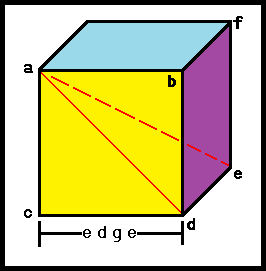Cube Area and Volume Calculator Scroll down for formulas, instructions and definitions.since "ac" and "cd" are "edges" then:
ad = edge • square root of (2)

face diagonal = edge • square root of (2)

Calculating the main diagonal "ae":

ae² = face diagonal af² + edge fe²
We already calculated the face diagonal, so we know:
ae² = [edge • square root of (2)]² + edge²
ae² = 2 • edge² + edge²
ae² = 3 • edge²
ae² = edge • square root of (3)

main diagonal = edge • square root of (3)

Total Cube Area = edge² • 6

Cube Volume = edge³

Edge Length = (Cube Volume)(⅓)

Calculator Instructions

This calculator is extremely easy to use. Merely input the length of 1 edge of the cube, click on "CALCULATE" and you'll have your answers.
The above figure is called a cube or a regular hexahedron.
A cube is one of the 5 platonic solids.
Lines ac = cd = db = ab and are called edges.
Each "corner" of a cube (points a, b, c and d for example) is called a vertex.
Each plane of a cube is a square (plane a b c d for example) and is called a face.
Every cube has 6 faces, 8 vertices and 12 edges.
Dice are good examples of cubes.

Significant Figures >>>
The default setting is for 5 significant figures but you can change that by inputting another number in the box above.

Answers are displayed in scientific notation and for easier readability, numbers between .001 and 1,000 will be displayed in standard format (with the same number of significant figures.)
The answers should display properly but there are a few browsers that will show no output whatsoever. If so, enter a zero in the box above. This eliminates all formatting but it is better than seeing no output at all.